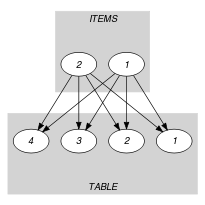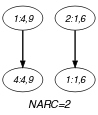## 5.146. elements

Origin
Constraint

$\mathrm{𝚎𝚕𝚎𝚖𝚎𝚗𝚝𝚜}\left(\mathrm{𝙸𝚃𝙴𝙼𝚂},\mathrm{𝚃𝙰𝙱𝙻𝙴}\right)$

Arguments
 $\mathrm{𝙸𝚃𝙴𝙼𝚂}$ $\mathrm{𝚌𝚘𝚕𝚕𝚎𝚌𝚝𝚒𝚘𝚗}\left(\mathrm{𝚒𝚗𝚍𝚎𝚡}-\mathrm{𝚍𝚟𝚊𝚛},\mathrm{𝚟𝚊𝚕𝚞𝚎}-\mathrm{𝚍𝚟𝚊𝚛}\right)$ $\mathrm{𝚃𝙰𝙱𝙻𝙴}$ $\mathrm{𝚌𝚘𝚕𝚕𝚎𝚌𝚝𝚒𝚘𝚗}\left(\mathrm{𝚒𝚗𝚍𝚎𝚡}-\mathrm{𝚒𝚗𝚝},\mathrm{𝚟𝚊𝚕𝚞𝚎}-\mathrm{𝚍𝚟𝚊𝚛}\right)$
Restrictions
 $\mathrm{𝚛𝚎𝚚𝚞𝚒𝚛𝚎𝚍}$$\left(\mathrm{𝙸𝚃𝙴𝙼𝚂},\left[\mathrm{𝚒𝚗𝚍𝚎𝚡},\mathrm{𝚟𝚊𝚕𝚞𝚎}\right]\right)$ $\mathrm{𝙸𝚃𝙴𝙼𝚂}.\mathrm{𝚒𝚗𝚍𝚎𝚡}\ge 1$ $\mathrm{𝙸𝚃𝙴𝙼𝚂}.\mathrm{𝚒𝚗𝚍𝚎𝚡}\le |\mathrm{𝚃𝙰𝙱𝙻𝙴}|$ $\mathrm{𝚛𝚎𝚚𝚞𝚒𝚛𝚎𝚍}$$\left(\mathrm{𝚃𝙰𝙱𝙻𝙴},\left[\mathrm{𝚒𝚗𝚍𝚎𝚡},\mathrm{𝚟𝚊𝚕𝚞𝚎}\right]\right)$ $\mathrm{𝚃𝙰𝙱𝙻𝙴}.\mathrm{𝚒𝚗𝚍𝚎𝚡}\ge 1$ $\mathrm{𝚃𝙰𝙱𝙻𝙴}.\mathrm{𝚒𝚗𝚍𝚎𝚡}\le |\mathrm{𝚃𝙰𝙱𝙻𝙴}|$ $\mathrm{𝚍𝚒𝚜𝚝𝚒𝚗𝚌𝚝}$$\left(\mathrm{𝚃𝙰𝙱𝙻𝙴},\mathrm{𝚒𝚗𝚍𝚎𝚡}\right)$
Purpose

All the items of $\mathrm{𝙸𝚃𝙴𝙼𝚂}$ should be equal to one of the entries of the table $\mathrm{𝚃𝙰𝙱𝙻𝙴}$.

Example
$\left(\begin{array}{c}〈\mathrm{𝚒𝚗𝚍𝚎𝚡}-4\mathrm{𝚟𝚊𝚕𝚞𝚎}-9,\mathrm{𝚒𝚗𝚍𝚎𝚡}-1\mathrm{𝚟𝚊𝚕𝚞𝚎}-6〉,\hfill \\ 〈\begin{array}{cc}\mathrm{𝚒𝚗𝚍𝚎𝚡}-1\hfill & \mathrm{𝚟𝚊𝚕𝚞𝚎}-6,\hfill \\ \mathrm{𝚒𝚗𝚍𝚎𝚡}-2\hfill & \mathrm{𝚟𝚊𝚕𝚞𝚎}-9,\hfill \\ \mathrm{𝚒𝚗𝚍𝚎𝚡}-3\hfill & \mathrm{𝚟𝚊𝚕𝚞𝚎}-2,\hfill \\ \mathrm{𝚒𝚗𝚍𝚎𝚡}-4\hfill & \mathrm{𝚟𝚊𝚕𝚞𝚎}-9\hfill \end{array}〉\hfill \end{array}\right)$

The $\mathrm{𝚎𝚕𝚎𝚖𝚎𝚗𝚝𝚜}$ constraint holds since each item of its first argument $\mathrm{𝙸𝚃𝙴𝙼𝚂}$ corresponds to an item of the $\mathrm{𝚃𝙰𝙱𝙻𝙴}$ collection: the first item $〈\mathrm{𝚒𝚗𝚍𝚎𝚡}-4\mathrm{𝚟𝚊𝚕𝚞𝚎}-9〉$ of $\mathrm{𝙸𝚃𝙴𝙼𝚂}$ corresponds to the fourth item of $\mathrm{𝚃𝙰𝙱𝙻𝙴}$, while the second item $〈\mathrm{𝚒𝚗𝚍𝚎𝚡}-1\mathrm{𝚟𝚊𝚕𝚞𝚎}-6〉$ of $\mathrm{𝙸𝚃𝙴𝙼𝚂}$ corresponds to the first item of $\mathrm{𝚃𝙰𝙱𝙻𝙴}$.

Typical
 $|\mathrm{𝙸𝚃𝙴𝙼𝚂}|>1$ $\mathrm{𝚛𝚊𝚗𝚐𝚎}$$\left(\mathrm{𝙸𝚃𝙴𝙼𝚂}.\mathrm{𝚒𝚗𝚍𝚎𝚡}\right)>1$ $|\mathrm{𝚃𝙰𝙱𝙻𝙴}|>1$ $\mathrm{𝚛𝚊𝚗𝚐𝚎}$$\left(\mathrm{𝚃𝙰𝙱𝙻𝙴}.\mathrm{𝚟𝚊𝚕𝚞𝚎}\right)>1$
Symmetries
• Items of $\mathrm{𝙸𝚃𝙴𝙼𝚂}$ are permutable.

• Items of $\mathrm{𝚃𝙰𝙱𝙻𝙴}$ are permutable.

• All occurrences of two distinct values in $\mathrm{𝙸𝚃𝙴𝙼𝚂}.\mathrm{𝚟𝚊𝚕𝚞𝚎}$ or $\mathrm{𝚃𝙰𝙱𝙻𝙴}.\mathrm{𝚟𝚊𝚕𝚞𝚎}$ can be swapped; all occurrences of a value in $\mathrm{𝙸𝚃𝙴𝙼𝚂}.\mathrm{𝚟𝚊𝚕𝚞𝚎}$ or $\mathrm{𝚃𝙰𝙱𝙻𝙴}.\mathrm{𝚟𝚊𝚕𝚞𝚎}$ can be renamed to any unused value.

Arg. properties

Functional dependency: $\mathrm{𝙸𝚃𝙴𝙼𝚂}.\mathrm{𝚟𝚊𝚕𝚞𝚎}$ determined by $\mathrm{𝙸𝚃𝙴𝙼𝚂}.\mathrm{𝚒𝚗𝚍𝚎𝚡}$ and $\mathrm{𝚃𝙰𝙱𝙻𝙴}$.

Usage

Used for replacing several $\mathrm{𝚎𝚕𝚎𝚖𝚎𝚗𝚝}$ constraints sharing exactly the same table by a single constraint.

Reformulation

The $\mathrm{𝚎𝚕𝚎𝚖𝚎𝚗𝚝𝚜}$$\left(〈\mathrm{𝚒𝚗𝚍𝚎𝚡}-{I}_{1}\mathrm{𝚟𝚊𝚕𝚞𝚎}-{V}_{1},\mathrm{𝚒𝚗𝚍𝚎𝚡}-{I}_{2}\mathrm{𝚟𝚊𝚕𝚞𝚎}-{V}_{2},\cdots ,\mathrm{𝚒𝚗𝚍𝚎𝚡}-{I}_{|\mathrm{𝙸𝚃𝙴𝙼𝚂}|}\mathrm{𝚟𝚊𝚕𝚞𝚎}-{V}_{|\mathrm{𝙸𝚃𝙴𝙼𝚂}|}〉,\mathrm{𝚃𝙰𝙱𝙻𝙴}\right)$ constraint can be expressed in term of a conjunction of $|\mathrm{𝙸𝚃𝙴𝙼𝚂}|$ $\mathrm{𝚎𝚕𝚎𝚖}$ constraints of the form:

$\mathrm{𝚎𝚕𝚎𝚖}$$\left(〈\mathrm{𝚒𝚗𝚍𝚎𝚡}-{I}_{1}\mathrm{𝚟𝚊𝚕𝚞𝚎}-{V}_{1}〉,\mathrm{𝚃𝙰𝙱𝙻𝙴}\right)$,

$\mathrm{𝚎𝚕𝚎𝚖}$$\left(〈\mathrm{𝚒𝚗𝚍𝚎𝚡}-{I}_{2}\mathrm{𝚟𝚊𝚕𝚞𝚎}-{V}_{2}〉,\mathrm{𝚃𝙰𝙱𝙻𝙴}\right)$,

$\cdots$

$\mathrm{𝚎𝚕𝚎𝚖}$$\left(〈\mathrm{𝚒𝚗𝚍𝚎𝚡}-{I}_{|\mathrm{𝙸𝚃𝙴𝙼𝚂}|}\mathrm{𝚟𝚊𝚕𝚞𝚎}-{V}_{|\mathrm{𝙸𝚃𝙴𝙼𝚂}|}〉,\mathrm{𝚃𝙰𝙱𝙻𝙴}\right)$.

Keywords
Cond. implications

$\mathrm{𝚎𝚕𝚎𝚖𝚎𝚗𝚝𝚜}\left(\mathrm{𝙸𝚃𝙴𝙼𝚂},\mathrm{𝚃𝙰𝙱𝙻𝙴}\right)$

with  $\mathrm{𝚍𝚒𝚜𝚝𝚒𝚗𝚌𝚝}$$\left(\mathrm{𝙸𝚃𝙴𝙼𝚂},\mathrm{𝚒𝚗𝚍𝚎𝚡}\right)$

and   $\mathrm{𝚃𝙰𝙱𝙻𝙴}.\mathrm{𝚟𝚊𝚕𝚞𝚎}\ge 0$

implies $\mathrm{𝚋𝚒𝚗}_\mathrm{𝚙𝚊𝚌𝚔𝚒𝚗𝚐}_\mathrm{𝚌𝚊𝚙𝚊}$$\left(\mathrm{𝚃𝙰𝙱𝙻𝙴},\mathrm{𝙸𝚃𝙴𝙼𝚂}\right)$.

Arc input(s)

$\mathrm{𝙸𝚃𝙴𝙼𝚂}$ $\mathrm{𝚃𝙰𝙱𝙻𝙴}$

Arc generator
$\mathrm{𝑃𝑅𝑂𝐷𝑈𝐶𝑇}$$↦\mathrm{𝚌𝚘𝚕𝚕𝚎𝚌𝚝𝚒𝚘𝚗}\left(\mathrm{𝚒𝚝𝚎𝚖𝚜},\mathrm{𝚝𝚊𝚋𝚕𝚎}\right)$

Arc arity
Arc constraint(s)
 $•\mathrm{𝚒𝚝𝚎𝚖𝚜}.\mathrm{𝚒𝚗𝚍𝚎𝚡}=\mathrm{𝚝𝚊𝚋𝚕𝚎}.\mathrm{𝚒𝚗𝚍𝚎𝚡}$ $•\mathrm{𝚒𝚝𝚎𝚖𝚜}.\mathrm{𝚟𝚊𝚕𝚞𝚎}=\mathrm{𝚝𝚊𝚋𝚕𝚎}.\mathrm{𝚟𝚊𝚕𝚞𝚎}$
Graph property(ies)
$\mathrm{𝐍𝐀𝐑𝐂}$$=|\mathrm{𝙸𝚃𝙴𝙼𝚂}|$

Graph model

Parts (A) and (B) of Figure 5.146.1 respectively show the initial and final graph associated with the Example slot. Since we use the $\mathrm{𝐍𝐀𝐑𝐂}$ graph property, the arcs of the final graph are stressed in bold.

##### Figure 5.146.1. Initial and final graph of the $\mathrm{𝚎𝚕𝚎𝚖𝚎𝚗𝚝𝚜}$ constraint(a) (b)
Signature

Since all the $\mathrm{𝚒𝚗𝚍𝚎𝚡}$ attributes of $\mathrm{𝚃𝙰𝙱𝙻𝙴}$ collection are distinct and because of the first condition $\mathrm{𝚒𝚝𝚎𝚖𝚜}.\mathrm{𝚒𝚗𝚍𝚎𝚡}=\mathrm{𝚝𝚊𝚋𝚕𝚎}.\mathrm{𝚒𝚗𝚍𝚎𝚡}$ of the arc constraint, a source vertex of the final graph can have at most one successor. Therefore $|\mathrm{𝙸𝚃𝙴𝙼𝚂}|$ is the maximum number of arcs of the final graph and we can rewrite $\mathrm{𝐍𝐀𝐑𝐂}=|\mathrm{𝙸𝚃𝙴𝙼𝚂}|$ to $\mathrm{𝐍𝐀𝐑𝐂}\ge |\mathrm{𝙸𝚃𝙴𝙼𝚂}|$. So we can simplify $\underline{\overline{\mathrm{𝐍𝐀𝐑𝐂}}}$ to $\overline{\mathrm{𝐍𝐀𝐑𝐂}}$.